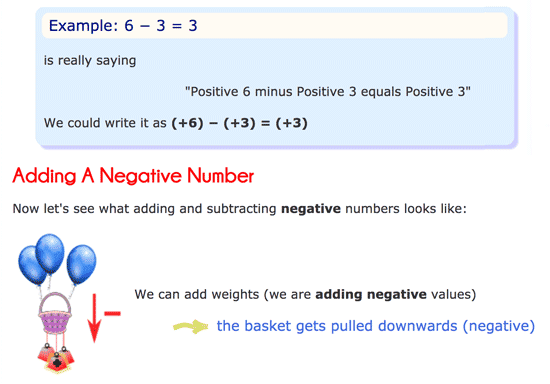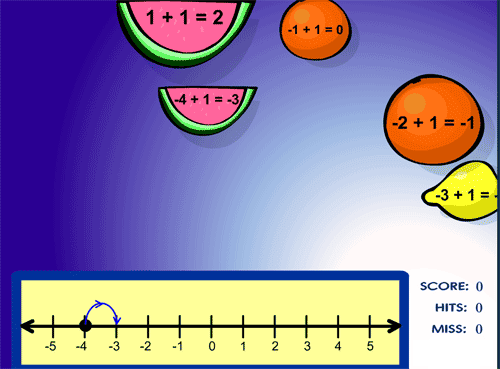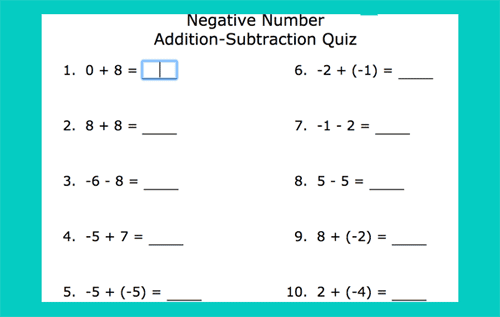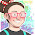## Tuesday, 19 February 2019

### Unit 7 - Negative numbers: addition and subtraction

Hello, hello!
In this Maths unit we're learning about negative numbers and we have to know how to add and subtract integers.Here you have an explanation:Here you have some exercises and games to practice addition and subtraction of whole numbers:**NEW**Negative numbers word problems. KS2 MathsCasey Runner, a game to practice subtracting and adding whole numbersFruit shoot integer addition gameFruit splat game with addition of whole numbersSubtracting negative numbers online exerciseSubtraction with negative numbersNegative numbers addition and subtractionNegative number addition-subtraction quizAdding and subtracting integer word problems

I hope you find these useful!
Bye, bye, butterflies!

1.Hello Remei! we have to do both diagrams subtracting a positive number and adding a negative number or only one?
PAU

1.Hello, Pau! Just the one on "Adding a negative number".
Keep up the good job!

2.hello remei.i am patri ,I have seen my work of arts in your instagram, thank you.also y have seen some of the videos of social sc,maths,and y have played some games of maths.But I have a question:why some games work and others tell me"this is not permitted"or something like that?